U.S. Department of Transportation
1200 New Jersey Avenue, SE
Washington, DC 20590
202-366-4000

Federal Highway Administration Research and Technology
Coordinating, Developing, and Delivering Highway Transportation Innovations

 REPORT This report is an archived publication and may contain dated technical, contact, and link information
 Publication Number:  FHWA-HRT-17-098     Date:  January 2018
 Publication Number: FHWA-HRT-17-098 Date: January 2018

# Self-Enforcing Roadways: A Guidance Report

## APPENDIX A. OPERATING SPEED EQUATIONS

Table 33. Equations from literature used to determine operating speeds.
Study Year No. Equation R2 Variables Units Conditions/Notes
Fitzpatrick et al. 2005 1 *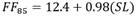0.904 SL Metric
Fitzpatrick et al. 2005 2 *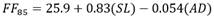0.923 SL, AD Metric For sites with posted speed limit of 45 mph (73 km/h) or less
Fitzpatrick et al. 2005 3 *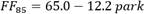0.50 park Metric For sites with 30 mph (48 km/h) posted speed limit
Misaghi and Hassan 2005 1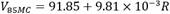0.464 R Metric
Misaghi and Hassan 2005 2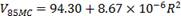0.524 R Metric
Misaghi and Hassan 2005 3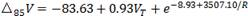0.649 R, VT Metric
Misaghi and Hassan 2005 4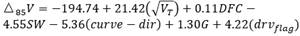0.889 VT, DFC, SW, curve direction, G, drvflag Metric
Fitzpatrick et al. 2003 1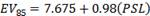0.904 PSL U.S. customary All roadways
Fitzpatrick et al. 2003 2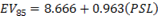0.86 PSL U.S. customary Suburban/urban arterial
Fitzpatrick et al. 2003 3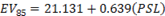0.41 PSL U.S. customary Suburban/urban collector
Fitzpatrick et al. 2003 4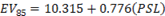0.14 PSL U.S. customary Suburban/urban local
Fitzpatrick et al. 2003 5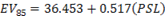0.81 PSL U.S. customary Rural arterial
Schurr et al. 2002 1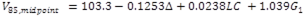0.46 Δ, LC, G1 Metric
Schurr et al. 2002 2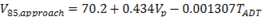0.19 Vp, TADT Metric
Schurr et al. 2002 3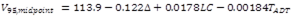0.41 Δ, LC, TADT Metric
Schurr et al. 2002 4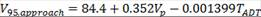0.22 Vp, TADT Metric
Fitzpatrick et al. 2000a 1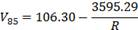0.92 R Metric Horizontal curve on grade: 0 ≤ G < 4 or horizontal curve combined with sag vertical curve
Fitzpatrick et al. 2000a 2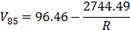0.56 R Metric Horizontal curve on grade: 4 ≤ G < 9
Fitzpatrick et al. 2000a 3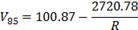0.59 R Metric Horizontal curve on grade:
-9 ≤ G< 0
Fitzpatrick et al. 2000a 4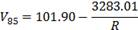0.78 R Metric Horizontal curve combined with limited sight distance crest vertical curve
Fitzpatrick et al. 2000a 5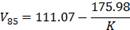0.54 K Metric Vertical crest curve with limited sight distance on horizontal tangents
Fitzpatrick et al. 2000a 6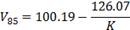0.68 K Metric Sag vertical curve on horizontal tangent
Polus et al. 2000 1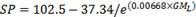0.332 GML Metric GML ≤ 200; maximum speed = 63.69 mph (102.5 km/h)
Polus et al. 2000 2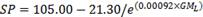0.228 GML Metric GML ≤ 1000; maximum speed = 65.25 mph (105 km/h)
Polus et al. 2000 30.553 GMS Metric Small radii and small TL; R≤ 250 m; TL < 150 m
Polus et al. 2000 4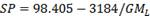0.684 GML Metric Small radii and intermediate TL; R ≤ 250 m; 150 m < TL < 1000 m; GML ≤ 1500
Polus et al. 2000 5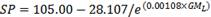0.742 GML Metric If maximum 85th-percentile speed is 65.25 mph (105 km/h)
Polus et al. 2000 6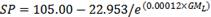0.838 GML Metric Large TL and any reasonable radius;
TL< 1000 m
Krammes et al. 1995 1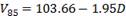0.80-0.82 D Metric 85th-percentile speed on long tangents overestimated
Krammes et al. 1995 2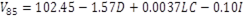0.82 D, LC, I Metric
Krammes et al. 1995 3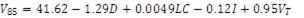0.90 D, LC, I, VT Metric
Lamm and Choueiri 1987 1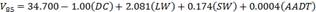[mph] 0.842 DC, LW, SW, AADT U.S. customary
Lamm and Choueiri 1987 2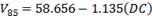[mph] 0.787 DC U.S. customary
Lamm and Choueiri 1987 3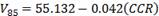[mph] 0.846 CCR U.S. customary Lane width = 10 ft
Lamm and Choueiri 1987 4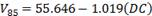[mph] 0.753 DC U.S. customary Lane width = 10 ft
Lamm and Choueiri 1987 5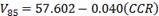0.731 CCR U.S. customary Lane width = 11 ft
Lamm and Choueiri 1987 6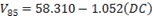0.746 DC U.S. customary Lane width = 11 ft
Lamm and Choueiri 1987 7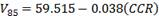0.836 CCR U.S. customary Lane width = 12 ft
Lamm and Choueiri 1987 8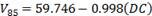0.824 DC U.S. customary Lane width = 12 ft
McLean 1979 1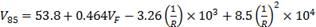0.92 VF, R Metric
Fitzpatrick et al. 1995 1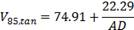0.71 AD Metric Suburban highways, tangent sections
Fitzpatrick et al. 1995 2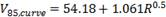0.72 R Metric Suburban highways, curve sections
Fitzpatrick et al. 1995 3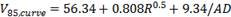0.81 R, AD Metric Suburban highways, curve sections
Banihashemi et al. 2011 1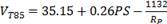0.49 RP U.S. customary Tangents with LT < 150 ft
Banihashemi et al. 2011 2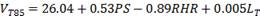0.29 PS, RHR, LT U.S. customary Tangents with LT ≥ 150 ft
Banihashemi et al. 2011 3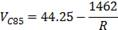0.37 R U.S. customary Curve speed

*©ASCE. Reproduced with the express written authority of the American Society of Civil Engineers (ASCE). Excerpted from the ASCE publication

Journal of Transportation Engineering, 131(4), ASCE, April 2005, pp. 261-269.

†©ASCE. Reproduced with the express written authority of the American Society of Civil Engineers (ASCE). Excerpted from the ASCE publication

Journal of Transportation Engineering, 131(4), ASCE, April 2005, pp. 408-418.

—No information.

FF85 = 85th-percentile free-flow operating speed (mph (km/h));
SL = posted speed limit (mph (km/h));
park = indicator variable for the presence of parking;
V85MC = 85th-percentile speed at middle of curve (mph (km/h));
Δ85V = 85th-percentile speed differential calculated as 85th-percentile value of speed differentials of individual drivers;
VT = approach tangent speed (mph (km/h));
DFC = deflection angle of circular curve (degrees);
SW = shoulder width (ft (m));
curve - dir = curve direction;
drvflag = driveway flag; EV85 = estimated 85th-percentile speed (mph (km/h));
PSL = posted speed limit (mph (km/h));
V85, midpoint = 85th-percentile speed at free-flow passenger cars at the curve midpoint (mph (km/h));
Δ = deflection or intersection angle of horizontal curve (degrees);
LC = length of horizontal curve (ft (m));
V85, approach = 85th-percentile speed at the approach location (mph (km/h));
Vp = posted speed limit (mph (km/h));
TADT = average daily traffic (vehicles per day);
V95, midpoint = 95th-percentile operating speed at the midpoint of the horizontal curve (mph (km/h));
V95, approach = 95th-percentile operating speed on the approach tangent to the horizontal curve (mph (km/h));
K = rate of vertical curvature (ft/percent (m/percent));
SP = posted speed limit (mph (km/h));
GML = geometric measure for long tangents between horizontal curves (ft2 (m2));
GMS = geometric measure for short tangents between horizontal curves (ft2 (m2));
D = degree of curvature;
I = deflection or intersection angle of horizontal curve (degrees);
LW = lane width (ft (m));
CCR = curvature change rate (degrees per half mile);
VF = desired speed of the 85th-percentile car (mph (km/h));
V85, tan = estimated 85th-percentile operating speed on tangent (mph (km/h));
V85, curve = 85th-percentile speed on the curve (mph (km/h));
VT85 = estimated 85th-percentile operating speed on tangent (mph (km/h));
PS = posted speed limit (mph (km/h));
Rp = radius of preceding horizontal curve (ft (m));